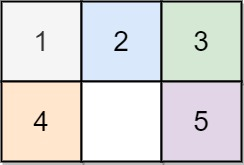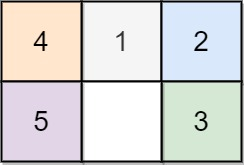## Algorithm

Problem Name: 773. Sliding Puzzle

On an `2 x 3` board, there are five tiles labeled from `1` to `5`, and an empty square represented by `0`. A move consists of choosing `0` and a 4-directionally adjacent number and swapping it.

The state of the board is solved if and only if the board is `[[1,2,3],[4,5,0]]`.

Given the puzzle board `board`, return the least number of moves required so that the state of the board is solved. If it is impossible for the state of the board to be solved, return `-1`.

Example 1:```Input: board = [[1,2,3],[4,0,5]]
Output: 1
Explanation: Swap the 0 and the 5 in one move.
```

Example 2:```Input: board = [[1,2,3],[5,4,0]]
Output: -1
Explanation: No number of moves will make the board solved.
```

Example 3:```Input: board = [[4,1,2],[5,0,3]]
Output: 5
Explanation: 5 is the smallest number of moves that solves the board.
An example path:
After move 0: [[4,1,2],[5,0,3]]
After move 1: [[4,1,2],[0,5,3]]
After move 2: [[0,1,2],[4,5,3]]
After move 3: [[1,0,2],[4,5,3]]
After move 4: [[1,2,0],[4,5,3]]
After move 5: [[1,2,3],[4,5,0]]
```

Constraints:

• `board.length == 2`
• `board[i].length == 3`
• `0 <= board[i][j] <= 5`
• Each value `board[i][j]` is unique.

## Code Examples

### #1 Code Example with C++ Programming

```Code - C++ Programming```

``````
class Solution {
public:
int slidingPuzzle(vector>& board) {
unordered_setvisited;
vector>dst({{1,2,3}, {4,5,0}});
string target = toString(dst);

int level = 0;

queue>>cur, next;

cur.push(board);

while(!cur.empty()) {
auto node = cur.front();
cur.pop();

string s = toString(node);

if (s == target) {
return level;
}

visited.insert(s);

auto nextStates = nextState(node);

for (auto v: nextStates) {
string t = toString(v);
if (!visited.count(t)) {
next.push(v);
}
}
if (cur.empty()) {
++level;
swap(cur, next);
}
}
return -1;
}

vector>> nextState(vector>& board) {
vector>>res;
auto pos = findZero(board);
int r = pos;
int c = pos;

int left  = c - 1;
int right = c + 1;
int up    = r - 1;
int down  = r + 1;

if (left >= 0) {
swap(board[r][left], board[r][c]);
res.push_back(board);
swap(board[r][left], board[r][c]);
}
if (right < 3) {
swap(board[r][right], board[r][c]);
res.push_back(board);
swap(board[r][right], board[r][c]);
}
if (up >= 0) {
swap(board[up][c], board[r][c]);
res.push_back(board);
swap(board[up][c], board[r][c]);
}
if (down < 2) {
swap(board[down][c], board[r][c]);
res.push_back(board);
swap(board[down][c], board[r][c]);
}
return res;
}

vector findZero(vector>& board) {
for (int i = 0; i < 2; ++i) {
for (int j = 0; j < 3; ++j) {
if (board[i][j] == 0) {
return {i, j};
}
}
}
}

string toString(vector>& v) {
string s;
for (auto x: v) {
for (auto y: x) {
s += to_string(y) + ",";
}
}
return s;
}
};
``````
Copy The Code &

Input

cmd
board = [[1,2,3],[4,0,5]]

Output

cmd
1

### #2 Code Example with Javascript Programming

```Code - Javascript Programming```

``````
const slidingPuzzle = function(board) {
const target = "123450";
let start = "";
for (let i = 0; i < board.length; i++) {
for (let j = 0; j < board.length; j++) {
start += board[i][j];
}
}
const visited = {};
// all the positions 0 can be swapped to
const dirs = [[1, 3], [0, 2, 4], [1, 5], [0, 4], [1, 3, 5], [2, 4]];
const queue = [];
queue.push(start);
visited[start] = true;
let res = 0;
while (queue.length !== 0) {
// level count, has to use size control here, otherwise not needed
let size = queue.length;
for (let i = 0; i < size; i++) {
let cur = queue.shift();
if (cur === target) {
return res;
}
let zero = cur.indexOf("0");
// swap if possible
for (let dir of dirs[zero]) {
let next = swap(cur, zero, dir);
if (visited.hasOwnProperty(next)) {
continue;
}
visited[next] = true;
queue.push(next);
}
}
res++;
}
return -1;
};

function swap(str, i, j) {
const arr = str.split("");
const ic = str[i];
const jc = str[j];
arr.splice(i, 1, jc);
arr.splice(j, 1, ic);
return arr.join("");
}
``````
Copy The Code &

Input

cmd
board = [[1,2,3],[4,0,5]]

Output

cmd
1

### #3 Code Example with Python Programming

```Code - Python Programming```

``````
class Solution:
def slidingPuzzle(self, board):
moves, used, cnt = {0: {1, 3}, 1:{0, 2, 4}, 2:{1, 5}, 3:{0, 4}, 4:{1, 3, 5}, 5:{2, 4}}, set(), 0
s = "".join(str(c) for row in board for c in row)
q = [(s, s.index("0"))]
while q:
new = []
for s, i in q:
if s == "123450":
return cnt
arr = [c for c in s]
for move in moves[i]:
new_arr = arr[:]
new_arr[i], new_arr[move] = new_arr[move], new_arr[i]
new_s = "".join(new_arr)
if new_s not in used:
new.append((new_s, move))
cnt += 1
q = new
return -1
``````
Copy The Code &

Input

cmd
board = [[1,2,3],[5,4,0]]

Output

cmd
-1

### #4 Code Example with C# Programming

```Code - C# Programming```

``````
using System;
using System.Collections.Generic;

namespace LeetCode
{
public class _0773_SlidingPuzzle
{
private (int dr, int dc)[] direction = new (int dr, int dc) { (1, 0), (-1, 0), (0, 1), (0, -1) };

public int SlidingPuzzle(int[][] board)
{
State final = new State(new int[]
{
new int[] { 1, 2, 3 },
new int[] { 4, 5, 0 },
});
State initState = new State(board);

Queue queue = new Queue();
ISet visited = new HashSet();

queue.Enqueue(initState);

int count = 0;
while (queue.Count > 0)
{
int size = queue.Count;
for (int i = 0; i < size; i++)
{
var curr = queue.Dequeue();
if (curr.Equals(final)) return count;

foreach (var dir in direction)
{
var next = curr.Move(dir);
if (next != null && visited.Add(next))
{
queue.Enqueue(next);
}
}
}

count++;
}

return -1;
}

private class State : IEquatable
{

public State(int[][] board)
{
_board = board;
}

public override bool Equals(object obj) => Equals(obj as State);

public override int GetHashCode()
{
unchecked
{
int res = 0;
for (int i = 0; i < 2; i++)
for (int j = 0; j < 3; j++)
{
res *= 10;
res += _board[i][j];
}
return res.GetHashCode();
}
}

public bool Equals(State other)
{
for (int i = 0; i < 2; i++)
for (int j = 0; j < 3; j++)
if (_board[i][j] != other._board[i][j])
return false;

return true;
}

private State Clone()
{
int[][] newBoard = new int[];
for (int i = 0; i < 2; i++)
{
newBoard[i] = new int;
for (int j = 0; j < 3; j++)
newBoard[i][j] = _board[i][j];
}

return new State(newBoard);
}

public State Move((int dr, int dc) dir)
{
for (int i = 0; i < 2; i++)
for (int j = 0; j < 3; j++)
if (_board[i][j] == 0)
{
int newI = i + dir.dr;
int newJ = j + dir.dc;

if (newI >= 0 && newI < 2 && newJ >= 0 && newJ < 3)
{
var tmp = _board[i][j];
_board[i][j] = _board[newI][newJ];
_board[newI][newJ] = tmp;

State res = Clone();

tmp = _board[i][j];
_board[i][j] = _board[newI][newJ];
_board[newI][newJ] = tmp;

return res;
}

return null;
}

return null;
}
}
}
}
``````
Copy The Code &

Input

cmd
board = [[1,2,3],[5,4,0]]

Output

cmd
-1